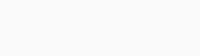Tuesday, December 5, 2023

# After setting up conversion tracking, you want to add a column for conversion value per cost. How would that be calculated?

-

After setting up conversion tracking, you want to add a column for conversion value per cost. How would that be calculated?

• It would be calculated by dividing the total conversion value by the total cost of all ad interactions.
• It would be calculated by dividing the total conversion value by the number in the conversions column.
• It would be calculated by dividing the total cost by the number in the conversions column.
• It would be calculated by dividing the number in the conversions column by the total eligible interactions.